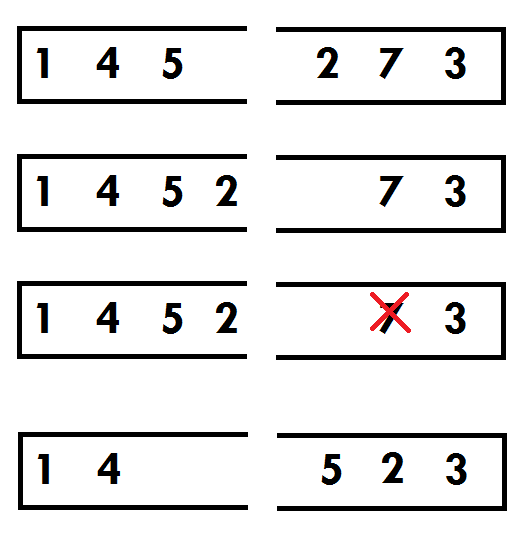# 洛谷 P3253 [JLOI2013]删除物品 题解【线段树】

## 题目描述

（1）一共有N个物品，堆成M堆。

（2）所有物品都是一样的，但是它们有不同的优先级。

（3）你只能够移动某堆中位于顶端的物品。

（4）你可以把任意一堆中位于顶端的物品移动到其它某堆的顶端。若此物品是当前所有物品中优先级最高的，可以直接将之删除而不用移动。

（5）求出将所有物品删除所需的最小步数。删除操作不计入步数之中。

（6）只是一个比较难解决的问题，这里你只需要解决一个比较简单的版本： 不会有两个物品有着相同的优先级，且M=2

3 3
1 4 5 2 7 3

6

## 说明

1<=N1+N2<=100000

根据题意，首先要把第一堆物品倒过来，这样就相当于两个栈对着放，其实这个题就是两个栈对跳的模拟，然而100000的数据让人不敢下手$O(N^2)$，因此我做的方法是计算当前修改的点到下一个需要修改的点的距离，同时还需要跳过已经被删除的点，就像这样（此处与样例有出入，不要看错了）我们首先将初始点定在5后面，然后计算删除7需要移动多少次，删除7后变成了1 4 5 2  |  3，此时再移动至1 4 5 | 2 3，计算方式是，如果下一个目标数字在栈口右侧，此时把可以不用付出代价的数字全部移动到左边来，也包含已经被删除的数字，这一过程结束后再计算代价，代价就是这两个点之间的数字个数减去这两个点之间被删除的数字个数。而我们可以用前缀和维护某点之前有多少个点被删除了，从而推导到每个点或每个区间。（前缀和按理说是树状数组的工作然而我只会线段树）当删除（例如）t点时，t之后的点都需要打上标记，即线段树的change(root,t,n,-1);，-1指的是前面删除了一个点。

不过看洛谷题解里有写维护区间剩余点的可能更好理解，不过因人而异吧。

这就是这段代码的核心部分，其中a[p].first>cnt意味着已经被删掉的点，移动这些点不需要代价。ask是线段树的查询函数，即前缀和，后一个减前一个是需要减掉的被取走元素的个数。

if(p>=pos[cnt])
{
while(a[p].first>cnt&&p>1)
p--;
}
else
{
p++;
while(a[p].first>cnt&&p<n)
p++;
}

同时，题面只强调了“数字越大，优先级越高”；“不会有两个物品有着相同的优先级”，但是没有说明优先级是否连续，甚至于是否很大的问题。因此我们可以使用离散化将n个数据映射到1~n里去。

最后对这个题有一点体会，下午一接触到这个题时，会想到差分数组维护这个前缀和，但是这个题实际上是在线的，因此不行。就想到了线段树，然而正解却始终没有打出来，看到还有半个小时下课，果断打了暴力，暴力改了几下是对的，于是暴力启发了我的解法的改进。可以说我的算法离标算还有点距离，但是毕竟用线段树优化了，就像是分块的特点“大段维护，局部朴素”，能做到这一点我想这是这道题除了自信以外所给我的最大收获了。

## Code:

#include<cstdio>
#include<iostream>
#include<algorithm>
#define ls (k<<1)
#define rs ((k<<1)|1)
#define mid ((l+r)>>1)
#define Mid ((t[k].l+t[k].r)>>1)
using std::pair;
using std::sort;
using std::max;
using std::min;
struct node
{
int l,r,v,lazy;
node(int l,int r)
{
this->l=l;
this->r=r;
v=0;
lazy=0;
}
node()
{
v=0,lazy=0;
}
}t;
pair<int,int> a;//离散化工具
bool cmp(pair<int,int> x,pair<int,int> y)
{
return x.second < y.second;
}
int pos;//存储每个数字（映射）所处的位置
void build(int k,int l,int r)//线段树建树
{
t[k]=node(l,r);
if(l==r)
return;
build(ls,l,mid);
build(rs,mid+1,r);
return;
}
void change(int k,int l,int r,int x)//线段树修改函数
{
if(t[k].l==l&&t[k].r==r)
{
t[k].lazy=t[k].lazy+x;
return;
}
if(t[k].lazy!=0)
{
t[k].v+=(t[k].r-t[k].l+1)*t[k].lazy;
if(t[k].l!=t[k].r)
{
t[ls].lazy+=t[k].lazy;
t[rs].lazy+=t[k].lazy;
}
t[k].lazy=0;
}
t[k].v+=(r-l+1)*x;
if(r<=Mid)
change(ls,l,r,x);
else if(l>Mid)
change(rs,l,r,x);
else
{
change(ls,l,Mid,x);
change(rs,Mid+1,r,x);
}
return ;
}
{
if(t[k].lazy!=0)
{
t[k].v+=(t[k].r-t[k].l+1)*t[k].lazy;
if(t[k].l!=t[k].r)
{
t[ls].lazy+=t[k].lazy;
t[rs].lazy+=t[k].lazy;
}
t[k].lazy=0;
}
if(t[k].l==l&&t[k].r==r)
return t[k].v;
if(r<=Mid)
else if(l>Mid)
else
}
int main()
{
long long ans=0;
int n1,n2,n;
scanf("%d%d",&n1,&n2);
for(int i=n1;i>=1;i--)
{
scanf("%d",&a[i].first);
a[i].second=i;
}
for(int i=n1+1;i<=n1+n2;i++)
{
scanf("%d",&a[i].first);
a[i].second=i;
}
n=n1+n2;
sort(a+1,a+n+1);
for(int i=1;i<=n;i++)
a[i].first=i;
sort(a+1,a+n+1,cmp);//离散过程
for(int i=1;i<=n;i++)
pos[a[i].first]=i;
build(1,1,n);
int cnt=n,p=n1,d;
//p相当于指针
while(cnt)
{
if(p>=pos[cnt])
{
while(a[p].first>cnt&&p>1)
p--;
}
else
{
p++;
while(a[p].first>cnt&&p<n)
p++;
}
ans+=d;
p=pos[cnt];
change(1,p,n,-1);
cnt--;
}
printf("%lld",ans);
return 0;
}


### 4 说点什么0 Followers

Most reacted comment
0 Comment authors
Recent comment authors
Subscribe[…] Find More to that Topic: wjyyy.top/169.html […][…] Information on that Topic: wjyyy.top/169.html […]wpDiscuz